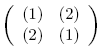occasional meanderings in physics' brave new world

## Monday, July 14, 2008

### M Theory Lesson 206

Carl Brannen's new post on 1-circulant and 2-circulant operators extends his previous analysis to the remainder of the fundamental fermions and their quantum numbers. He works with $6 \times 6$ circulants of the formfor $(1)$ a 1-circulant and $(2)$ a 2-circulant. Just as for the $2 \times 2$ case with numerical matrix entries, we can think of $(1) \pm (2)$ as the eigenvalues of the $6 \times 6$ operator. Notice that the idempotents obtained have simple 2-circulants $(2)$ of democratic form, which means that adding or subtracting them from $(1)$ results in another 1-circulant. For example, for the $e_{R}^{+}$ quantum numbers one finds thatwhich is a unitary 1-circulant since all entries have norm $\frac{1}{3}$. The same matrix results from $(1) + (2)$ for $\overline{\nu}_{R}$. The democratic matrix with all values equal to $\frac{1}{3}$ comes from, for instance, the $\overline{d}_{L}$ quark idempotent. Tony Smith, who likes to think of the Higgs as a top quark condensate, might like this correspondence between Higgs numbers and quark operators.CarlBrannen said...